Journal of Complexity in Health Sciences

Published: 30 June 2020

# Brain CT image segmentation based on 3D slicer

Yuxuan Wang1
Han Wang2
Keqin Shen3
Jincai Chang4
Jianzhong Cui5
1, 2, 3, 4College of Sciences, North China University of Science and Technology, Tangshan, 063210, China
5Department of Neurosurgery, Tangshan Gongren Hospital, Tangshan, 063000, China
1, 2, 3, 43D Modeling and Application Innovation Laboratory, North China University of Science and Technology, Tangshan, 063210, China
Corresponding Author:
Jincai Chang
Corresponding Author:
Jianzhong Cui
Views 1322

#### Abstract

This article focuses on CT images of human brain. Based on the characteristics of CT, 3D Slicer software was used to segment the brain CT images. First, the functions and features of 3D Slicer software are briefly introduced. Second, the principles of threshold segmentation and FCM algorithm are described. Using the Segment Editor module of 3D Slicer software to perform image segmentation, the threshold segmentation method and FCM algorithm are compared.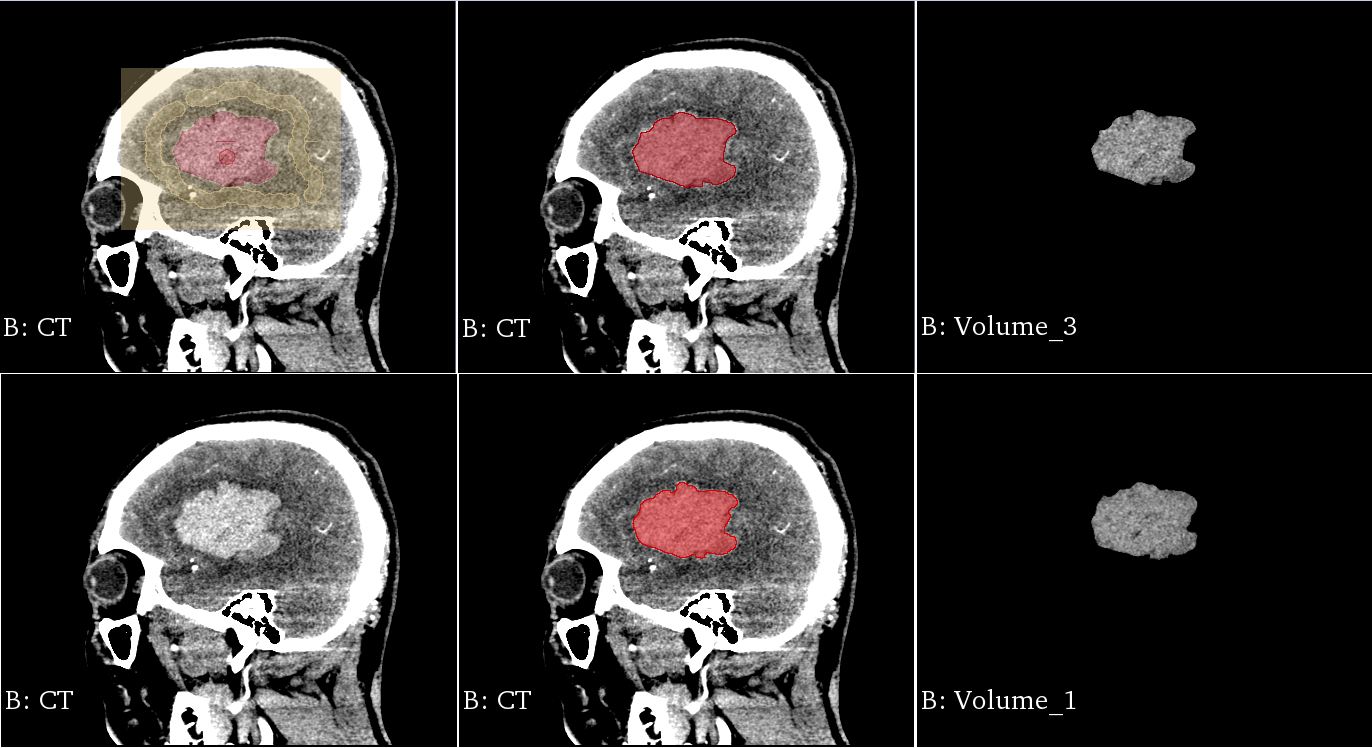#### Highlights

• The segmentation of human brain CT image is studied in 3D slicer, a medical image processing platform.
• Practical operation of image segmentation in Segment Editor module.
• Threshold segmentation method and Fuzzy c-means algorithm.

## 1. Introduction

### 1.1. Introduction of 3D slicer

3D Slicer  is a free open source software package based on VTK, ITK, CTK, KWW, CMake, Qt and Python. Its application scope is mainly the analysis and research of medical image, aiming at realizing the visualization of medical image. According to the principle of C++ programming Slicer code, ITK and VTK design method is also applied to it, MATLAB, Python, Tcl and other design code will also appear in it. C++ and python are its main programming languages. Slicer has a complex structure, but it has good learning value. The advantage of Slicer is its powerful function. Only when we have a detailed understanding of Slicer's structure can we understand and learn this complex and powerful system in depth.

### 1.2. Main features of 3D Slicer

(1) Multi-image format: It can transform the format of images to each other. Only in the work of filtering module can we do this work. The visualization function of 3D Slicer can be transformed by multiple data.

(2) Many functional compilers: a) DICOM module for reading images; b) Editor module for editing images; c) 3D subroutine drawing, perfect segmentation and fusion of surface information pixels; d) interactive visual compiler for image; e) several modules for geometric pixel segmentation; f) image fusion and registration; g) tracking of image bootstrap; h) other analysis; Module and so on.

(3) Dynamic loaded modules can organize Slicer's various functions. Slicer now supports three modules, and users see the same module. Slicer has different internal access rights for the three modules, and the developer chooses which module to use depends on the situation.

(4) The entity of translating and interpreting language provides ontology to an abstract object of other interfaces. The operation separates the external communication method so that it can be modified internally without affecting the way other entities interact with it. 3D Slicer can be adopted or encapsulated across platforms, and enables the 3D slicer platform to be used under Windows, Linux and other system platforms. It integrates many interfaces of biomedical research software communication, so it has good software compatibility.

## 2. The significance of medical image segmentation

(1) Obtain pathological information. Extract information about anatomical structures, lesions, conditions, movements, etc., to establish a database of anatomical structures for clinical diagnosis, pathological measurement;

(2) Measure characteristic parameters. Measure the geometric, physical, pathological, statistical and other characteristic parameters of each anatomical structure, and establish an anatomical structure information database;

(3) Locate the area of interest. Determine the location, shape, and distribution of the lesion structure, and locate targets for treatment options such as puncture and surgical image-guided surgery.

(4) Manage image information. After the image is segmented, each area is clear, and you can choose between them, focusing on those target areas. It can also compress image data without losing useful information, which is convenient for archiving, retrieval and transmission .

## 3. Current development of image segmentation

At present, there are many kinds of image segmentation algorithms, each of which can be used for image segmentation with certain characteristics. Image segmentation can usually be divided into two kinds. One is region-based image segmentation algorithm, which mainly uses the similarity of a certain feature attributes within the image area to segment different regions. Its representative methods are threshold segmentation and region growth method. The other is edge detection-based image segmentation algorithm, which mainly uses each region in the image. For the difference of some features, the concept of gradient is used to detect the edge and segment the boundary of different regions, mainly based on Canny operator algorithm and Sobel operator algorithm. With the development of specific theories in related fields in recent years, relevant theories have also been applied to image segmentation. Such as statistical theory, neural network theory, fuzzy theory, wavelet transform theory, morphological theory, etc., and began to consider image segmentation from a new perspective.

No matter which segmentation algorithm has its disadvantages and advantages. People put forward the concept of integrated segmentation technology, which combines more than two segmentation algorithms for image segmentation. Experiments show that the segmentation effect of the integrated segmentation algorithm is better than that of single segmentation algorithm. Therefore, the integration of various image segmentation algorithms is also an important direction of research. In addition, the medical image itself has features of boundary blur, uncertainty and excessive detail. The segmentation of medical images becomes more difficult, but this makes the fuzzy technology have room for use. The application of fuzzy theory to image segmentation is an important research direction in image segmentation. According to the latest research and trend, we mainly study image segmentation from three aspects. One is the evaluation method and evaluation criteria of image segmentation algorithm. The other is focused on the research of image segmentation algorithm itself. The third is the evaluation method of image segmentation algorithm.

There are various image segmentation algorithms based on various principles, but there are few real medical images. In addition, the medical image segmentation is complicated because of the excessive details, blurred boundary of brain tissue and irregular shape in CT images of craniocerebral injury. Research on automatic segmentation or semi-automatic segmentation to replace manual segmentation is an important goal of segmentation algorithm, which has important clinical significance .

## 4. Introduction to segment editor module in 3D slicer

Segment Editor module is a module that can be used for segmentation, subdivision (also known as outline) depicting interesting structure. Some tools mimic painting interfaces such as Photoshop or gimp, but can work on three-dimensional array voxels rather than two-dimensional pixels. Some new functions have been added to the new module editor formed after the module editor module has been improved. Including alternating subdivision, display in 2D and 3D views, visualization options for each segment, 3D view editing, creating segmentation by interpolation or interpolation on several slices, editing slices in any direction.

## 5. Segmentation of lesions in brain CT in 3D Slicer

### 5.1. The basic principle of threshold segmentation

Threshold segmentation  is a simple region-based image segmentation technique. Image pixel points are divided into several parts by setting different feature thresholds. Common features include: grayscale and color features directly from the original image. The transformed feature can effectively compress the amount of data, and can simplify the analysis and processing steps. Therefore, in general, the image preprocessing process is a necessary process before image analysis, feature extraction and pattern recognition. The purpose of image thresholding is to divide the pixel set according to the gray level. Each subset obtained forms an area corresponding to the real scene. Each area has the same attributes inside, and the adjacent area layout has the same attributes.

The global threshold method assumes that the image is basically composed of two parts, the object and the background. The simplest example is the object image from the background image. The most commonly used threshold segmentation method is to take advantage of the difference in gray level between the object and its background in the image. The image is regarded as a combination of two regions with different gray levels . And select an appropriate threshold to determine whether each pixel in the image should belong to the target area or the background area, so as to generate the corresponding binary image.

The basic principle of thresholding segmentation is described as follows:

1

In the formula, $Z$ is the threshold, $f\left(x,y\right)$ is any gray-scale geometry within the gray-scale range of the image, $E\in \left[{E}_{1},{E}_{K}\right]$${E}_{1}$, ${E}_{K}$, is the gray-scale of arbitrarily selected target and background. Identifying the target from a complex background and extracting it completely, selecting the threshold is a key step in the threshold segmentation technique.

In almost all medical images, both the object and the background have a distribution of gray values, and sometimes double or multi-peak in the gray histogram. By using the weighted transformed histogram, the peak-to-valley boundary more obvious than the original histogram can be obtained, thereby improving the image segmentation.

Different thresholds will produce different segmentation results. Generally speaking, we hope that the smiling change of the threshold does not cause a great change in the area or perimeter of the object to be segmented. That is, the segmentation result is insensitive to the change of the threshold. If $T$ is used to represent the threshold, $A\left(T\right)$ and $P\left(T\right)$ represent the area and perimeter of the segmented object respectively. If the selected threshold ${T}^{*}$ satisfies the following formula, ${T}^{*}$ is called the optimal threshold:

2

Gray-level histogram  is the two-dimensional relationship between the number of gray-level pixels ${n}_{i}$ and gray-level $i$. It reflects the statistical characteristics of gray-level distribution on an image.

If the histogram of a gray image has a gray level range of 0, 1,…, $l-1$ and the number of pixels is ${n}_{J}$ when the gray level is $J$, then the total pixel $N$ of an image is:

3
$N=\sum _{i=0}^{l-1}{n}_{i}={n}_{0}+{n}_{1}+\cdots +{n}_{l-1}.$

The probability of occurrence of gray level $i$ is:

4
${p}_{i}=\frac{{n}_{i}}{N}=\frac{{n}_{i}}{{n}_{0}+{n}_{1}+\cdots +{n}_{l-1}}.$

When the image in the grayscale image is relatively simple and the gray distribution of objects is regular, the peaks and peaks of the background and image are formed on the grayscale histogram of the image. Therefore, the gray value corresponding to the valley between the two peaks is chosen as the threshold value to separate the two regions.

The method of segmenting the target and background by thresholding histogram is called histogram threshold bimodal method. The implementation of the method is to first make the histogram of the image $f\left(x,y\right)$. If only the background and the corresponding histogram of the two regions and parts correspond to the obvious valley, the corresponding gray value of the bottom point can be taken as the threshold $t$. Then the segmentation based on the threshold can separate the target from the image .

### 5.2. Fuzzy c-means algorithm

Brain CT images are irregular, uncertain and fuzzy, and it is difficult to find clear boundaries between different tissues. The FCM clustering algorithm is suitable for the characteristics of uncertainty and ambiguity in gray images. It is an unsupervised fuzzy clustering process that reduces human intervention. When used for brain CT image segmentation, the FCM algorithm clusters the gray values of each pixel .

The FCM algorithm is as follows:

5
${J}_{m}\left(U,V\right)=\sum _{j=1}^{n}\sum _{i=1}^{c}\left({u}_{ij}{\right)}^{m}\left({d}_{ij}{\right)}^{2}.$

Eq. (5) is the clustering objective function, where $U=\left\{{u}_{ij}\right\}$ is the fuzzy membership matrix. ${u}_{ij}$ represents the degree of membership of the $j$-th data corresponding to the $i$-th category. $V=\left\{{v}_{i}\right\}$ is the set of cluster centers. ${d}_{ij}=‖{x}_{j}-{v}_{i}‖$ represents the distance from the $j$-th pixel to the $i$-th cluster center, and $c$ is the number of cluster categories, and $1. $m$ is the fuzzy weighted index, and $1\le m<\infty$. Set $X=\left\{{x}_{1},{x}_{2},\cdots ,{x}_{n}\right\}$ is a set of $n$ pixels to be clustered. The objective function ${J}_{m}$ is minimized by the following iterative optimization algorithm. The specific algorithm is as follows:

Step 1. Determine the parameters $c$, $m$, $\epsilon >$ 0, $t=$ 1, and set the initial cluster center arbitrarily ${V}^{\left(t\right)}$.

Step 2. Calculate the following formula:

6

When ${d}_{ij}=0$, then ${u}_{ij}=1$, ${u}_{kj}=0$, $k\ne i$, $j=1,2,\cdots ,n$.

Step 3. Calculate the new cluster center ${V}^{\left(t+1\right)}$:

7
${v}_{i}^{\left(t+1\right)}=\frac{\sum _{j=1}^{n}\left({{u}_{ij}}^{\left(t\right)}{\right)}^{m}{x}_{j}}{\sum _{j=1}^{n}\left({{u}_{ij}}^{\left(t\right)}{\right)}^{m}}.$

Step 4. If $‖{V}^{\left(t+1\right)}-{V}^{\left(t\right)}‖\le \epsilon$, stop iteration, otherwise let $t=t+1$, return step 2 .

### 5.3. Image segmentation in 3D slicer

(1) Import data: enter the Segment Editor module.

Fig. 1Select the segment editor module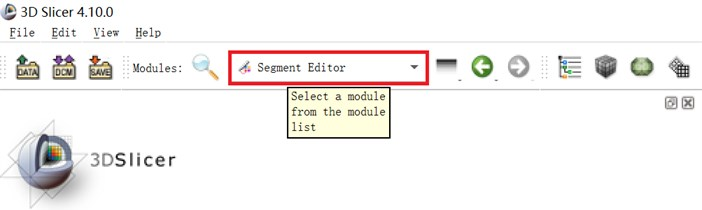(2) Definition level: define an editable segmentation structure.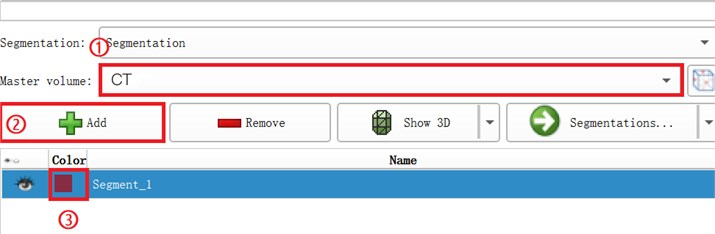(3) Adjust the parameters: enter the Threshold Effect setting option, adjust the threshold range, and make the threshold staining range completely cover the segmentation area .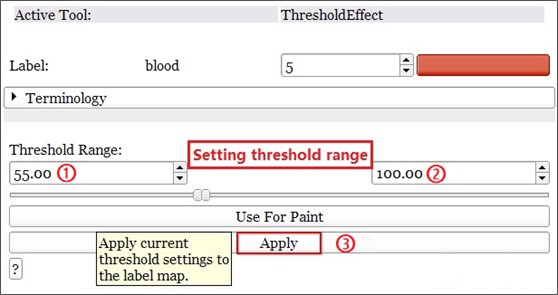(4) Select the target image: Use the islands function to separate the part to be separated from many small blocks. Select the function of keep select island in islands and click on the part to be separated. Selected image, this will be the segmented sett.

Fig. 4Separation operation by Islands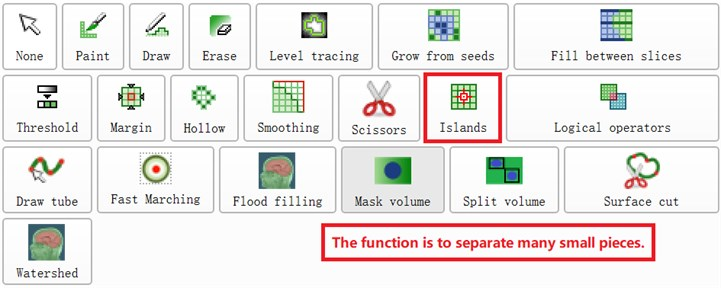(5) Run division: Use the Mask volume function to output the division and save the set parameters and run the program to get divided objects.

Fig. 5Segmentation and derivation of selected CT images by Mask volume module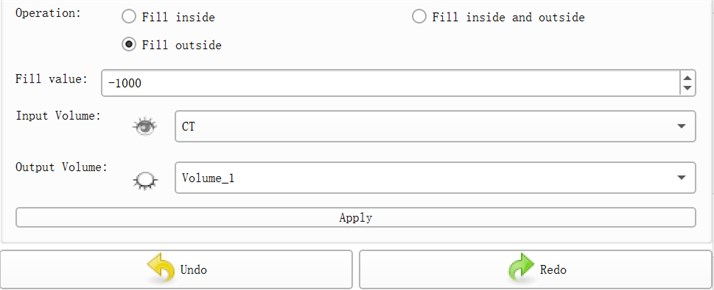### 5.4.1. Threshold segmentation method

Fig. 6 is the experimental data of CT brain hematoma images. The threshold method is used to achieve image segmentation, and the results are shown in the figure .

Fig. 6Image threshold segmentation results in the transverse section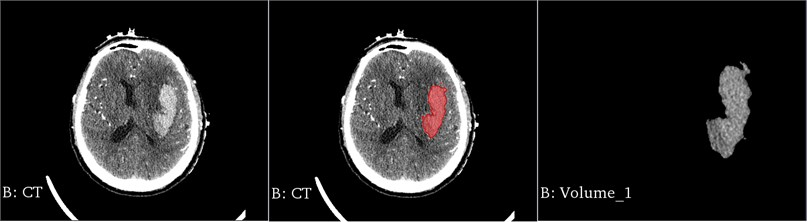Fig. 7Image threshold segmentation results on median sagittal section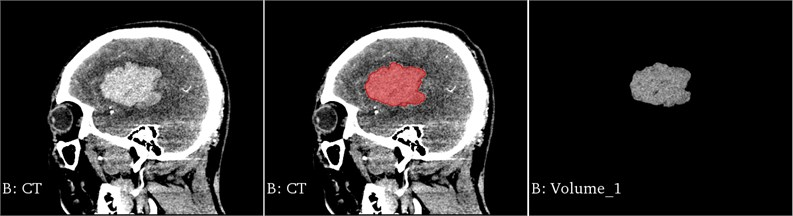Threshold segmentation has the best effect on CT slices, and the algorithm is simple and fast. However, when selecting the threshold, you need to judge based on experience, or do multiple tentative segmentation first, and then perform threshold adjustment.

### 5.4.2. Fuzzy c-means algorithm

The FCM algorithm has a long calculation time. For areas with smooth edges, it requires less interaction times to complete the segmentation. If the edge smoothness is poor, in order to perform accurate segmentation, the number of interactions must be increased, and the total time of segmentation will also be increased. This method can obtain better image segmentation results, a lot of detailed information is retained, and the edge area is also very clear, which is closer to the real block area .

Fig. 8Segmentation results of FCM algorithm in transverse section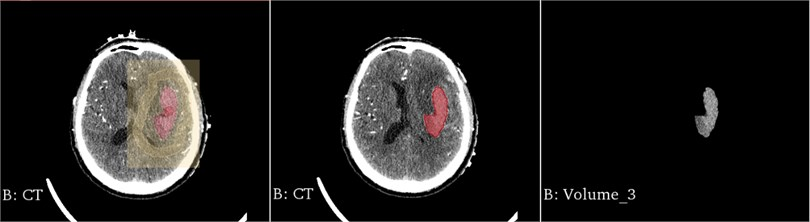Fig. 9Segmentation results of the FCM algorithm on the median sagittal section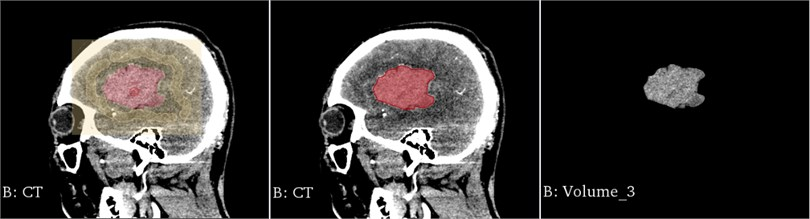## 6. Conclusion and outlook

Image segmentation is a very important content in image processing technology, and also a key link in image engineering. The quality of image segmentation directly affects the subsequent development of related work such as image analysis and image understanding. Among the many image segmentation methods, the threshold method is the most widely used and studied, and has the characteristics of low computational complexity and good segmentation effect. This paper deeply analyzes and discusses the principle and process of segmentation of medical image threshold method in the segment editor module of 3D Slicer, which provides a reference direction for future research.

This is a new field, and there are new directions waiting for us to explore. Many new technologies are waiting for us to master. There is still a lot of room for development in this area. Advancing with the times is our pursuit at this stage. And 3D Slicer is a software platform with great potential, it is worthy of us to study it in depth, and more to apply it in medical image processing analysis .

#### Acknowledgements

This work was supported by the National Science Foundation of China (51674121, 61702184), Tangshan Innovation Team Project (18130209B, 18130210B), the Hebei Key Laboratory of Science and Application, Research and practice project of higher education teaching reform in Hebei Province (2017GJJG111) and Ministry of education production university cooperation education project (201802305012).

#### Cited by

3D Printing of Heart Model as Medical Education Tools
2023 International Symposium on Medical Robotics (ISMR)
2022 7th International Conference on Signal and Image Processing (ICSIP)
Six artificial intelligence paradigms for tissue characterisation and classification of non-COVID-19 pneumonia against COVID-19 pneumonia in computed tomography lungs
2021 IEEE Applied Imagery Pattern Recognition Workshop (AIPR)
Quality Inspection of Gluing in Narrow Border Based on Depth Map